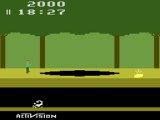Sam Trenholme's webpage
Support this website# Pitfall’s polynomial

## June 12 2013

In this blog, I continue looking at Pitfall's random number generator. This continues a series with two previous blog entries.

==Implementing an LFSR in 6502==

Here is one way of implementing a LFSR in 6502 and c:

uint8_t lfsr_1(uint8_t r) {
uint8_t a, c, t;
a = r;              // LDA r
c = a & 1; a >>= 1; // LSR;   a: r >> 1
c = a & 1; a >>= 1; // LSR;   a: r >> 2
a ^= r;             // EOR r; a: r >> 2 ^ r
c = a & 1; a >>= 1; // LSR;   a: r >> 3 ^ r >> 1
a ^= r;             // EOR r; a: r >> 3 ^ r >> 2 ^ r
c = a & 1; a >>= 1; // LSR;   a: r >> 4 ^ r >> 2 ^ r >> 1
a ^= r;             // EOR r; a: r >> 4 ^ r >> 2 ^ r >> 1 ^ r
c = a & 1; a >>= 1; // LSR;   a: r >> 5 ^ r >> 3 ^ r >> 2 ^ r >> 1
c = a & 1; a >>= 1; // LSR;   a: r >> 6 ^ r >> 4 ^ r >> 3 ^ r >> 2
c = a & 1; a >>= 1; // LSR;   a: r >> 7 ^ r >> 5 ^ r >> 4 ^ r >> 3
c = a & 1; a >>= 1; // LSR; c: (r >> 7 ^ r >> 5 ^ r >> 4 ^ r >> 3) & 1
t = r; r <<= 1; r |= (c & 1); c = t >> 7; // ROL r; r <<=1; r |= c;
return r;
}

The 6502 above is a bit confusing to follow because our code is affecting both the 8-bit accumulator (“a”) and a 1-bit carry flag (“c”). Until the final LSR, the accumulator is the variable affecting the final result; the final LSR is there to store the low bit of the accumulator in the carry flag. The subsequent “ROL r” operation shifts “r” one bit to the left, setting the low bit to the value of the carry flag.

Here is easier to follow equivalent code:

uint8_t lfsr_2(uint8_t random) {
uint8_t t; // temp
t = (random >> 7) ^ (random >> 5) ^ (random >> 4) ^ (random >> 3);
random <<= 1;
random |= t & 1;
return random;
}

When you need to store an entire game in 4,096 bytes, it is very important to make code as compact as possible. The above 6502 takes 18 bytes of code to render (“LDA r”, “EOR r”, and “ROL r” take two bytes; “LSR” uses one byte). Is there a way to make it smaller?

==More to come==

In a future blog entry, we will look at how to make the above code even smaller, and look at the code used in the actual Pitfall game.

Edit: The code I posted had an error (fixed June 16, 2013)